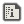# Drawing Ellipses on SmileBASIC```DEF ELLIPSE X,Y,SX,SY
VAR R,I,R2
FOR I=0TO 360:R2=R
GLINE X+SX*COS(R),Y+SY*SIN(R),X+SX*COS(R2),Y+SY*SIN(R2)
NEXT
END```
This basically has the same function that ellipse() does in processingJS, just ported to SmileBASIC.It would be faster to store the old X and Y coords so you don't have to recalculate SIN and COS every time.If you replace this line:
`GLINE X+SX*COS(R),Y+SY*SIN(R),X+SX*COS(R2),Y+SY*SIN(R2)`
With this line:
`GTRI X+SX*COS(R),Y+SY*SIN(R),X,Y,X+SX*COS(R2),Y+SY*SIN(R2)`
then it will draw a filled ellipse. c:Replying to:12Me21
It would be faster to store the old X and Y coords so you don't have to recalculate SIN and COS every time.
True, this is just something I made It's not exactly perfectReplying to:amihart
If you replace this line:
`GLINE X+SX*COS(R),Y+SY*SIN(R),X+SX*COS(R2),Y+SY*SIN(R2)`
With this line:
`GTRI X+SX*COS(R),Y+SY*SIN(R),X,Y,X+SX*COS(R2),Y+SY*SIN(R2)`
then it will draw a filled ellipse. c:
omfg, that's amazingReplying to:amihart
If you replace this line:
`GLINE X+SX*COS(R),Y+SY*SIN(R),X+SX*COS(R2),Y+SY*SIN(R2)`
With this line:
`GTRI X+SX*COS(R),Y+SY*SIN(R),X,Y,X+SX*COS(R2),Y+SY*SIN(R2)`
then it will draw a filled ellipse. c:
It would probably be faster just to use GPAINT, but then you'd have to do some extra things in case the ellipse had other stuff behind it, which might make it slower.```DEF ELLIPSE X,Y,SX,SY
VAR R#
VAR C#=SX,S#=0
FOR R#=0 TO 6.2831853 STEP 0.01 'or something
VAR OC#=C#
VAR OS#=S#
C#=SX*COS(R#)
S#=SY*SIN(R#)
GLINE X+OC#,Y+OS#,X+C#,Y+S#
NEXT
END
```
or somethingReplying to:12Me21
```DEF ELLIPSE X,Y,SX,SY
VAR R#
VAR C#=SX,S#=0
FOR R#=0 TO 6.2831853 STEP 0.01 'or something
VAR OC#=C#
VAR OS#=S#
C#=SX*COS(R#)
S#=SY*SIN(R#)
GLINE X+OC#,Y+OS#,X+C#,Y+S#
NEXT
END
```
or something
can you do a benchmark perhaps and compare it using MILLISEC? I lost my SD card therefore my access to SmileBASICReplying to:12Me21
```DEF ELLIPSE X,Y,SX,SY
VAR R#
VAR C#=SX,S#=0
FOR R#=0 TO 6.2831853 STEP 0.01 'or something
VAR OC#=C#
VAR OS#=S#
C#=SX*COS(R#)
S#=SY*SIN(R#)
GLINE X+OC#,Y+OS#,X+C#,Y+S#
NEXT
END
```
or something
It's significantly faster (if you adjust it to use the same number of steps), and my new version is over 2x as fast:
```DEF ELLIPSE X,Y,SX,SY
VAR R#
VAR C#=SX,S#=0
FOR R#=0 TO PI()/2+PI()*2/360 STEP PI()*2/360
VAR OC#=C#
C#=SX*COS(R#)
S#=SY*SIN(R#)

GLINE X+OC#,Y+OS#,X+C#,Y+S#
GLINE X-OC#,Y-OS#,X-C#,Y-S#
GLINE X-OC#,Y+OS#,X-C#,Y+S#
GLINE X+OC#,Y-OS#,X+C#,Y-S#
NEXT
END```
yours: 299 per sec. mine: 392 per sec. new: 741 per sec.Replying to:amihart
If you replace this line:
`GLINE X+SX*COS(R),Y+SY*SIN(R),X+SX*COS(R2),Y+SY*SIN(R2)`
With this line:
`GTRI X+SX*COS(R),Y+SY*SIN(R),X,Y,X+SX*COS(R2),Y+SY*SIN(R2)`
then it will draw a filled ellipse. c:
I made it so you can add #TRUE or #FALSE to the end of the function to make it filled or not.I have noticed something with this code.WHILE loops are faster than FOR loops.
`FOR`
example: I wrote this code to test the milliseconds it took to execute the following
```M=MILLISEC
FOR I=0 TO 1000000
'Just let it loop
NEXT
PRINT MILLISEC-M
'Returned approximately 750.
I=0'reset the counter to 0
M=MILLISEC
WHILE I<1000001
INC I
WEND
PRINT MILLISEC-M
```Replying to:DFrost
I have noticed something with this code.WHILE loops are faster than FOR loops.
`FOR`
example: I wrote this code to test the milliseconds it took to execute the following
```M=MILLISEC
FOR I=0 TO 1000000
'Just let it loop
NEXT
PRINT MILLISEC-M
'Returned approximately 750.
I=0'reset the counter to 0
M=MILLISEC
WHILE I<1000001
INC I
WEND
PRINT MILLISEC-M